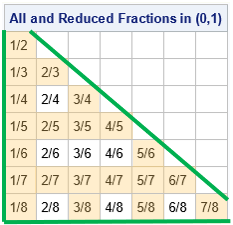Here is an interesting math question: How many reduced fractions in the interval (0, 1) have a denominator less than 100? The question is difficult is because of the word "reduced." If we only care about the total number of fractions in (0,1) whose denominator is less than 100, we could simply count the number of integer pairs (p,q), where 1 ≤ p < q < 100. For example, the table at the right shows all fractions whose denominators are less than 6. The green triangle encloses all n(n+1)/2 fractions in (0,1) for n=6. But only the fractions that have a gold background are in reduced form.

You can answer the question by generating a Farey sequence, which is the ordered set of reduced fractions (in reduced form) on the closed interval [0,1]. The Farey sequence has a surprising number of applications in pure and applied math. I first encountered it while studying the dynamics near resonant frequencies of forced coupled oscillators.

### The Farey sequence of order n

The Farey sequence is defined for fractions on the closed interval [0,1] and includes the endpoints as the reduced fractions 0/1 and 1/1. The Farey sequence of order n is the sequence of all reduced fractions on [0,1] whose denominators do not exceed n, listed in increasing order.

One algorithm for generating a Farey sequence would be to mimic the table at the top of this article. That is, generate all fractions with denominators up to n, then use a "sieve" to exclude fractions for which the numerator and denominator have a common factor. You would then have to sort the final set of results by the size of the fractions.

In fact, there is a better algorithm. The Wikipedia article about Farey sequences describes the algorithm as "surprisingly simple." The algorithm uses the "mediant property" of the Farey sequence to generate successive terms in order. The mediant property is the name given to the fact that each term in a Farey sequence is the mediant of its neighbors. Given two reduced fractions a/b and c/d, the mediant is the fraction (a+c)/(b+d). The mediant is sometimes called "freshman addition" (or, less kindly, "fool's addition") because the formula looks like a common mistake that children make when they learn to add fractions.

You can use the mediant property to generate the Farey sequence of order n from the Farey sequence of order n-1. However, as the Wikipedia article explains, you can also use the property to generate the Farey sequence of order n directly. The following DATA step generates the Farey sequences of order n for n ≤ 25.

```/* Farey sequence: http://en.wikipedia.org/wiki/Farey_sequence For each n, generate the Farey sequence of order n. This algorithm uses the mediant property of the Farey sequence to generate the terms. */ %let MaxOrder = 25; data Farey; do n = 1 to &MaxOrder; /* for each n, generate Farey sequence of order n */ Term=1; Numer = 0; Denom = 1; output; Term=2; Numer = 1; Denom = n; output; a=0; b=1; c=1; d=n; /* fractions are a/b and c/d */ do while (d > 1); Term = Term + 1; k = floor((n + b)/d); /* integer division */ a_prev = a; b_prev = b; a = c; b = d; /* current c/d becomes new a/b */ c = k*c - a_prev; d = k*d - b_prev; /* new c/d */ Numer = c; Denom = d; output; end; end; keep n Term Numer Denom; label n="Order"; run;```

Let's print out the terms of the Farey sequence of order n=8. The output shows the 21 fractions in the table at the top of this article, plus the trivial fractions 0/1 and 1/1 that are part of every Farey sequence. Notice the use of a DATA step view to compute the decimal values for the fractions, and the use of the FRACTw. format to display the decimals as fractions in their reduced form:

```data Fractions / view=Fractions; set Farey; Fraction = Numer / Denom; run;   proc print data=Fractions noobs; where n=8; format Fraction FRACT32.; run;```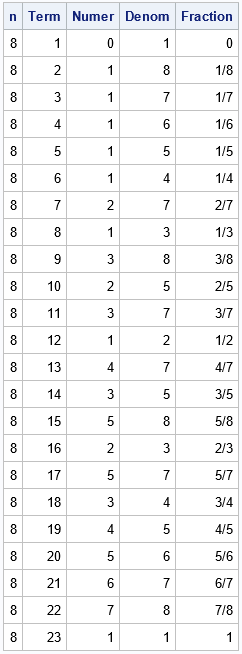### Visualize the Farey sequences

The Wikipedia article does not display my favorite visualization of the Farey sequences. It is interesting to plot the Farey sequence for order n for many values of n, as follows:

```ods graphics / width=500px height=400px; Title "Farey Sequences: Order 1 to 40"; proc sgplot data=Fractions; where n <= 40; scatter x=Fraction y=n / markerattrs=(symbol=CircleFilled) transparency=0.75; yaxis reverse grid integer values=(1, 5 to 40 by 5); xaxis grid display=(nolabel) values=(0 to 1 by 0.1); run;```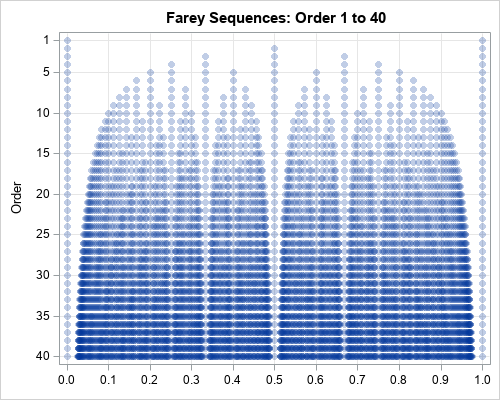The graph shows that there is a gap around each rational number in the Farey sequence. It turns out that the width of the gap (for each n) is larger for fractions that appear earlier in the Farey sequence. That is, if you look at the width of the gaps around 0 and 1, they are larger than the gap around 1/2, which is larger than the gaps around 1/3 and 2/3, and so forth. This turns out to be important in certain applications.

### The length of Farey sequences

In the previous graph, you can see that there are two markers on the first row, three on the second row, five on the third, and so forth. You can use PROC MEANS to find out how many terms are in the Farey sequence of order n. The following statements display the number of terms for n ≤ 10:

```proc means data=Farey noNObs nolabels N; where n <= 10; class n; var n; run;```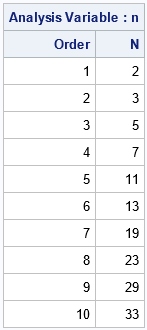You can use this technique to answer the question at the top of this article: how many reduced fractions in the interval (0, 1) have a denominator less than 100? The length of the Farey sequence of order 99 answers the question. You can use PROC MEANS to find the length of the Farey sequence of order 99:

```proc means data=Farey noNObs nolabels N; where n = 99; var n; run;```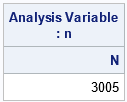There are 3005 terms in the Farey sequence of order 99, which includes the fractions 0/1 and 1/1. If you exclude the endpoints, you get the answer to the question: there are 3003 reduced fractions in the interval (0,1) that have a denominator less than 100.